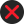Tax on income of Foreign Institutional Investors from securities or capital gains arising from their transfer. 115AD.   (1) Where the total income of a Foreign Institutional Investor includes- (a)  income 3{other than income by way of dividends referred to in section 115-O }received in respect of securities (other than units referred to in section 115AB); or (b)  income by way of short-term or long-term capital gains arising from the transfer of such securities, the income-tax payable shall be the aggregate of- (i)  the amount of income-tax calculated on the income in respect of securities referred to in clause (a), if any, included in the total income, at the rate of twenty per cent; Provided that the amount of income-tax calculated on the income by way of interest referred to in section 194LD shall be at the rate of five per cent; (ii)  the amount of income-tax calculated on the income by way of short-term capital gains referred to in clause (b), if any, included in the total income, at the rate of thirty per cent : Provided that the amount of income-tax calculated on the income by way of short-term capital gains referred to in section 111A shall be at the rate of fifteen per cent; (iii)  the amount of income-tax calculated on the income by way of long-term capital gains referred to in clause (b), if any, included in the total income, at the rate of ten per cent; 1[****] 2[Provided that in case of income arising from the transfer of a long-term capital asset referred to in section 112A, income-tax at the rate of ten per cent shall be calculated on such income exceeding one lakh rupees; and] (iv)  the amount of income-tax with which the Foreign Institutional Investor would have been chargeable had its total income been reduced by the amount of income referred to in clause (a) and clause (b). (2) Where the gross total income of the Foreign Institutional Investor- (a)  consists only of income in respect of securities referred to in clause (a) of sub-section (1), no deduction shall be allowed to it under sections 28 to 44C or clause (i) or clause (iii) of section 57 or under Chapter  VI-A; (b)  includes any income referred to in clause (a) or clause (b) of sub-section (1), the gross total income shall be reduced by the amount of such income and the deduction under Chapter VI-A shall be allowed as if the gross total income as so reduced, were the gross total income of the Foreign Institutional Investor. (3) Nothing contained in the first and second provisos to section 48 shall apply for the computation of capital gains arising out of the transfer of securities referred to in clause (b) of sub-section (1). Explanation.-For the purposes of this section,- (a)  the expression "Foreign Institutional Investor" means such investor as the Central Government may, by notification in the Official Gazette, specify in this behalf; (b)  the expression "securities" shall have the meaning assigned to it in clause (h) of section 2 of the Securities Contracts (Regulation) Act, 1956 (42 of 1956). Note- 1.Word "and" omitt. by the Finance Act, 2018 (w.e.f. 1-4-2019). 2. Ins. by the Finance Act, 2018, (w.e.f. 1-4-2019). 3. - Amendment Effective From 01st April, 2021